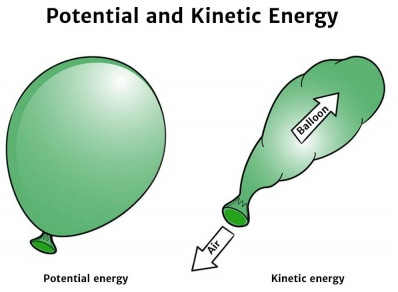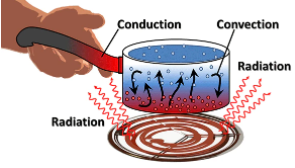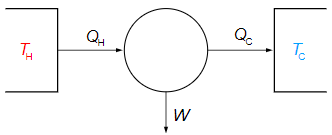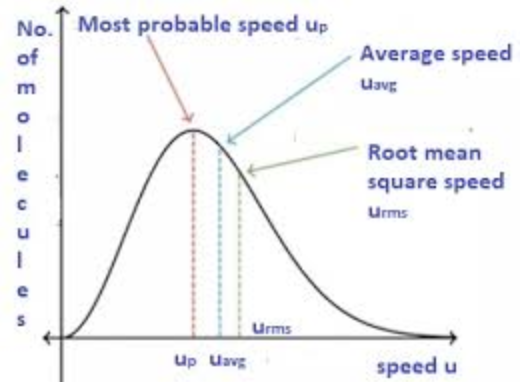# Class 11 Physics Case Study Questions## myCBSEguide App

CBSE, NCERT, JEE Main, NEET-UG, NDA, Exam Papers, Question Bank, NCERT Solutions, Exemplars, Revision Notes, Free Videos, MCQ Tests & more.

Looking for complete and comprehensive case study questions for class 11 Physics? myCBSEguide is just a click away! With extensive study materials, sample papers, case study questions and mock tests, myCBSEguide is your one-stop solution for class 11 Physics exam preparation needs. So, what are you waiting for? Log on to myCBSEguide and get started today!

## What is the purpose of physics?

Physics is the study of the fundamental principles governing the natural world. It is a vital part of the scientific enterprise, providing the foundation on which other sciences are built. Physics is essential for understanding how the world works, from the smallest particles to the largest structures in the Universe. In class 11 Physics, students are introduced to the basic concepts of physics and learn about the fundamental principles governing the natural world. Class 11 Physics concepts are essential for understanding the world around us and for further study in physics and other sciences.## What are case study questions in physics?

In physics, case study questions are intended to evaluate a student’s ability to apply theoretical principles to real-life situations. These questions usually ask the student to assess data from a specific experiment or setting in order to discover what physical principles are at play. Problem-solving and critical-thinking skills are developed through case study questions, which are an important aspect of physics education.

## CBSE Case Study Questions in Class 11 Physics

CBSE Class 11 Physics question paper pattern includes case study questions. Class 11 Physics case study questions assess a student’s ability to apply physics principles to real-world environments. The questions are usually focused on a situation provided in the Class 11 Physics question paper, and they demand the student to answer the problem using their physics knowledge. Class 11 Physics case study questions are an important aspect of the CBSE physics curriculum. Class 11 Physics case study questions are a useful way to assess a student’s expertise in the subject.

## Sample Class 11 Physics Case Study Questions

Expert educators at myCBSEguide have created a collection of Class 11 physics case study questions. The samples of Class 11 physics case study questions are given below. Class 11 physics case study questions are designed to test your understanding of the concepts and principles of physics. They are not meant to be easy, but they should be done if you have a good grasp of the subject. So, take a look at the questions and see how you fare. Good luck!

### Class 11 Physics Case Study Question 1

Potential energy is the energy stored within an object, due to the object’s position, arrangement or state. Potential energy is one of the two main forms of energy, along with kinetic energy. Potential energy depends on the force acting on the two objects.1. A body is falling freely under the action of gravity alone in vacuum. Which of the following quantities remain constant during the fall?
1. kinetic energy
2. potential energy
3. mechanical energy
4. none of these
2. Work done by a conservative force is positive, if
1. potential energy decreases
2. potential energy increases
3. kinetic energy decreases
4. kinetic energy increases
3. When does the potential energy of a spring increases?
1. only when spring is stretched
2. only when spring is compressed
3. both a and b
4. none of these
4. Dimension of k/m is, here k is force constant
1. T2
2. T-2
3. T1
4. T-1
5. A vehicle of mass 5000kg climbs up a hill of 10 m. The potential energy gained by it
1. 5 J
2. 500 J
3. × 104 J
4. × 105 J

1. c
2. a
3. c
4. b
5. d

### Class 11 Physics Case Study Question 2

The figure shows the different modes of transfer of heat, heat transfer is defined as the movement of heat across the border of the system due to a difference in temperature between the system and its surroundings. The temperature difference exists between the two systems, heat will find a way to transfer from the higher to the lower system.1. The sea breeze is caused by:
1. conduction
2. convection
4. none of these
2. At what factor heat absorbed on radiation by the body depends on?
1. distance between body
2. source of heat
3. its color
4. all of the above
3. When heat is transferred by molecular collision, it is referred to as heat transfer by:
1. convection
2. conduction
4. Thermal conductivity of air with rise in temperature:
1. increase
2. decrease
3. constant
4. none of these
5. Mass transfer does not take place in-
1. conduction
2. convection
4. none of these

1. (b) convection
2. (d) all of the above
3. (a) convection
4. (a) increase

### Class 11 Physics Case Study Question 3

Carnot principles are only for the cyclical devices like heat engines, which state that the efficiency of an irreversible heat engine is always less than the efficiency of a reversible one operating between the same two reservoirs. The efficiencies of all reversible heat engines operating between the same two reservoirs are the same.1. In a Carnot cycle, the working medium rejects heat at a ________ temperature.
1. Higher
2. Lower
3. constant
4. none of these
2. Which of the following is NOT a state variable?
1. work
2. internal energy.
3. entropy
4. all of the above
3. The efficiency of reversible heat engine is:
1. 1 +(T2/T1)
2. (T1/T2)+1
3. (T1 /T2)- 1
4. 1 – (T2 / T1)
4. Other factors remaining constant, if the temperature of the source is increased, the efficiency of the Carnot engine will:
1. decrease
2. increase
3. constant
4. increase or decrease depending upon temperature ratio
5. Over the complete Carnot cycle, entropy:
1. increase
2. decrease
3. constant
4. first increase and then decrease

1. (b) lower
2. (a) Work
3. (d) 1- (T2/ T1)
4. (b) increase
5. (c) constant

### Class 11 Physics Case Study Question 4

Root mean square velocity (RMS value)is the square root of the mean of squares of the velocity of individual gas molecules and the Average velocity is the arithmetic mean of the velocities of different molecules of a gas at a given temperature.1. Moon has no atmosphere because:-
1. It is far away from the surface of the earth
2. Its surface temperature is 10°C
3. The r.m.s. velocity of all the gas molecules is more than the escape velocity of the moon’s surface
4. The escape velocity of the moon’s surface is more than the r.m.s velocity of all molecules
2. For an ideal gas, CPCVis
1. >1
2. <1
3. ≤1
4. none of these
3. The root mean square velocity of hydrogen is 5 times than that of nitrogen. If T is the temperature of the gas then:
1. T(H2) = T(N2)
2. T(H2) < T(N2)
3. T(H2) > T(N2)
4. none of these
4. Suppose the temperature of the gas is tripled and N2 molecules dissociate into an atom. Then what will be the rms speed of atom:
1. v06
2. v0
3. v03
4. none of these
5. The velocities of the molecules are v, 2v, 3v, 4v & 5v. The rms speed will be:
1.  11 v
2.  v(11)12
3.  v
4.  v(12)11

1. (c)
2. (b)
3. (b)
4. (a)
5. (b)

The given samples of Class 11 Physics case study questions will help Class 11 Physics students to get an idea on how to solve it. These Class 11 Physics case study questions are based on the topics covered in the Class 11 Physics syllabus and are designed to test the student’s conceptual understanding. The questions are of varying difficulty levels and cover a wide range of topics. By solving these Class 11 Physics case study questions, students will be able to develop their problem-solving skills and improve their understanding of the concepts.

## Examining Class 11 Physics syllabus

Senior Secondary school education is a transitional step from general education to a discipline-based curriculum concentration. The current curriculum of Class 11 Physics takes into account the rigour and complexity of the disciplinary approach, as well as the learners’ comprehension level. Class 11 Physics syllabus has also been carefully crafted to be similar to international norms.

The following are some of the Class 11 Physics syllabus’s most notable features:

1. Emphasis is placed on gaining a fundamental conceptual knowledge of the material.
2. Use of SI units, symbols, naming of physical quantities, and formulations in accordance with international standards are emphasised.
3. For enhanced learning, provide logical sequencing of subject matter units and suitable placement of concepts with their links.
4. Eliminating overlapping concepts/content within the field and between disciplines to reduce the curricular load.
5. Process skills, problem-solving ability, and the application of Physics principles are all encouraged.

CBSE Class 11 Physics (Code No. 042)

 No. of Periods Marks Unit–I Physical World and Measurement 08 23 Chapter–2: Units and Measurements Unit-II Kinematics 24 Chapter–3: Motion in a Straight Line Chapter–4: Motion in a Plane Unit–III Laws of Motion 14 Chapter–5: Laws of Motion Unit–IV Work, Energy and Power 14 17 Chapter–6: Work, Energy and Power Unit–V Motion of System of Particles and Rigid Body 18 Chapter–7: System of Particles and Rotational Motion Unit-VI Gravitation 12 Chapter–8: Gravitation Unit–VII Properties of Bulk Matter 24 20 Chapter–9: Mechanical Properties of Solids Chapter–10: Mechanical Properties of Fluids Chapter–11: Thermal Properties of Matter Unit–VIII Thermodynamics 12 Chapter–12: Thermodynamics Unit–IX Behaviour of Perfect Gases and Kinetic Theory of Gases 08 Chapter–13: Kinetic Theory Unit–X Oscillations and Waves 26 10 Chapter–14: Oscillations Chapter–15: Waves Total 160 70

## myCBSEguide: A true saviour for many students

myCBSEguide has been a true saviour for many students who struggle to find resources elsewhere. It is a reliable source of information and provides students with everything they need to excel in their academics. myCBSEguide has helped many students score high marks in their exams and has been a valuable resource for their studies. Teachers recommend myCBSEguide to all CBSE students. And best of all, it’s available 24/7, so you can study at your own pace, anytime, anywhere. So why wait? Get started today and see the difference myCBSEguide can make to your studies.